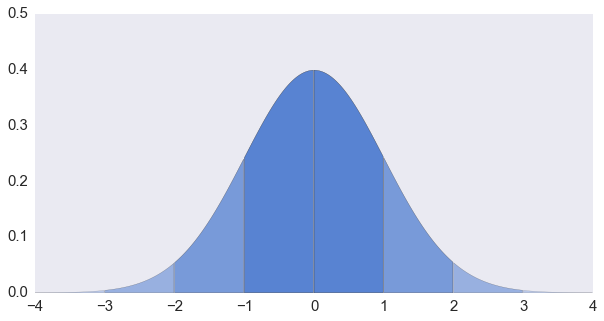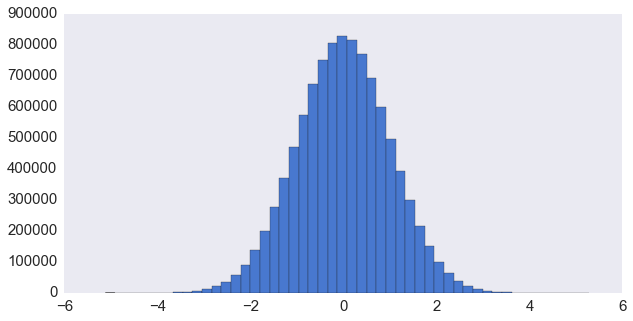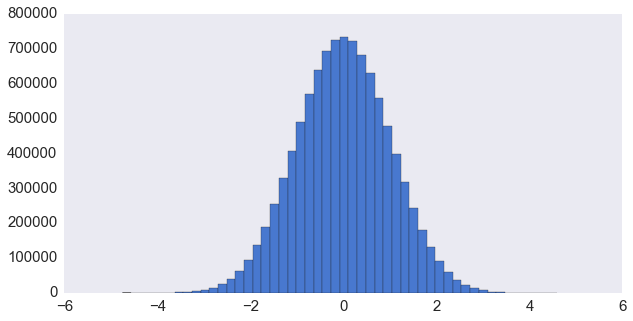# 正态分布随机数的生成 (1)

$$f(x \; | \; \mu, \sigma) = \frac{1}{\sigma\sqrt{2\pi} } \; e^{ -\frac{(x-\mu)^2}{2\sigma^2} }$$## 反变换法

import numpy as np
import scipy.stats as stats
import matplotlib.pyplot as plt
N = 10 ** 7
%time x = stats.norm.ppf(np.random.rand(N, 1))
plt.hist(x, 50)
Wall time: 1.58 sx = stats.norm.cdf(x)

## 中心极限定理……还是不要用的好

$$Z = \frac{\displaystyle\sum_{i=1}^n X_i – E\left(\displaystyle\sum_{i=1}^n X_i\right)}{\sqrt{\mathrm{Var}\left(\displaystyle\sum_{i=1}^n X_i\right)}}= \frac{\displaystyle\sum_{i=1}^n X_i – \frac{n}{2}}{\sqrt{n} \sqrt{\frac{1}{12}}} \sim N(0,1).$$

$$Z = \sum_{i=1}^{12} U_i – 6 \sim N(0,1).$$

%time g = np.sum(np.random.rand(N, 12), 1) - 6
plt.hist(g, 50)
Wall time: 2.55 sstats.normaltest(g)
NormaltestResult(statistic=4785.7373110266581, pvalue=0.0)

stats.normaltest(np.sum(np.random.rand(1000, 12), 1) - 6)
NormaltestResult(statistic=1.8167274769305835, pvalue=0.40318339808171011)

## Box-Muller 变换

$$I = \int_{-\infty}^{\infty} e^{-\frac{x^2}{2}} \mathrm{d} x$$

$$I^2 = \int_{-\infty}^{\infty} e^{-\frac{x^2}{2}} \mathrm{d} x \int_{-\infty}^{\infty} e^{-\frac{y^2}{2}} \mathrm{d} y = \int_{-\infty}^{\infty} \int_{-\infty}^{\infty} e^{-\frac{x^2+y^2}{2}} \mathrm{d} x \, \mathrm{d} y$$

$$\mathrm{d}x\,\mathrm{d}y = \begin{vmatrix}\frac{\partial x}{\partial r} & \frac{\partial x}{\partial \theta} \\ \frac{\partial y}{\partial r} & \frac{\partial y}{\partial \theta} \end{vmatrix} \mathrm{d}r\,\mathrm{d}\theta= r\, \mathrm{d}r\,\mathrm{d}\theta$$

$$I ^2 = \int_{r=0}^{\infty}\int_{\theta=0}^{2\pi}e^{-\frac{r^2}{2}} r\,\mathrm{d}r\,\mathrm{d}\theta = 2\pi\int_{r=0}^{\infty}e^{-\frac{r^2}{2}} r\,\mathrm{d}r = 2\pi\int_{r=0}^{\infty}e^{-\frac{r^2}{2}} \mathrm{d}\left(\frac{r^2}{2}\right) =2\pi$$

$$\Theta = 2\pi U_1$$

$$\mathbb{P}(R\leq r) = \int_{r’=0}^r e^{-\frac{r’^2}{2}}\,r’\,\mathrm{d}r’ = 1- e^{-r^2/2}$$

$$R = \sqrt{-2\ln(U_2)}$$

Python 里面的 random.gauss() 函数用的就是这样一个实现。我们来试试看：

%time x = [random.gauss(0, 1) for _ in range(N)]
Wall time: 10.4 s

%time x = np.sqrt(-2 * np.log(np.random.rand(N, 1))) * np.cos(2 * np.pi * np.random.rand(N, 1))
Wall time: 736 ms

\begin{align} X &= R \cos(\Theta) =\sqrt{-2 \ln U_1} \cos(2 \pi U_2)\\ Y &= R \sin(\Theta) =\sqrt{-2 \ln U_1} \sin(2 \pi U_2) \end{align}

$$f_{U_1,U_2}(u,v) = \frac{1}{\pi}$$

$$f_{R,\Theta}(r, \theta) = \frac{r}{\pi}$$

$\Theta$ 是均匀分布在 $[0, 2\pi)$ 上的，所以

$$f_R(r) = \int_0^{2\pi} f_{R,\Theta}(r, \theta)\,\mathrm{d}\theta = 2r$$

$$f_{R^2}(s) = f_R(r) \frac{\mathrm{d}r}{\mathrm{d}(r^2)} = 2r \cdot \frac{1}{2r} = 1$$

$$\cos \Theta, \sin\Theta = \frac{U_1}{R}, \frac{U_2}{R} = \frac{U_1}{\sqrt{s}}, \frac{U_2}{\sqrt{s}}$$

$$u\sqrt{\frac{-2\ln s}{s}}, v\sqrt{\frac{-2\ln s}{s}}$$

%time x = np.random.randn(N)
Wall time: 363 ms